Sunday 20th September 2020

CBSE Guess > Papers > Question Papers > Class XII > 2003 > Mathematics > Compartment Delhi Set -I

MATHEMATICS—2003 (Set I—Compartment Delhi)

SECTION - A

Q. 1. Show that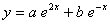is a solution of the differential equation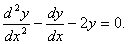Q. 2. Solve the x and y, given thatQ. 3. Evaluate:Q. 4. Evaluate;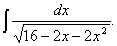Q. 5. Evaluate: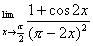Q. 6. Using properties of determinants, prove the following: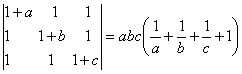Q. 7. If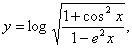find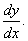Or

If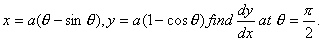Q. 8. Solve the following differential equation: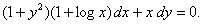Or

Solve the following differential equation: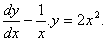Q. 9. Evaluate: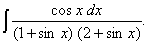Q. 10. Prove that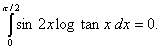Or

Prove that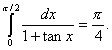Q. 11. If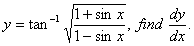Q. 12. Events A and B are given to be independent. Find P (B), if it is given that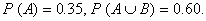Q. 13. Prove that the curves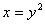and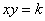cut each other at right angles if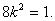Q. 14. The two lines of regression are given byFind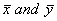and the co-efficient of correlation between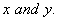Q. 15. A and B throw a die alternately till one of them gets a "6" and wins the games. Find their respective probability of winning if A starts the games.

Or

The probability of A solving a problem is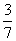and that of B solving it is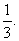What is the probability that
(i) At lest one of them will solve the problem?
(ii) Only one of them will solve the problem?

Q. 16. Using integration, find the area of the triangle ABC, Whose vertices have coordinates: A (2, 0), B (4, 5) and C (6, 3).

Q. 17. Prove that the volume of the largest cone be inscribed in a sphere of radius R is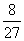of the volume of the sphere.

Q. 18. Given that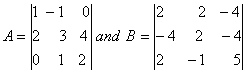. Find AB.
Use this to solve the following system of equations: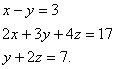SECTION - B

Q. 19. Find the value of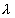so that the two vectors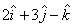and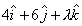are
(i) Parallel
(ii) Perpendicular to each other:

Q. 20. Find the foot of the perpendicular from the point (0, 2, 3) on the line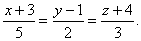Also fine the length of the perpendicular:

Q. 21. Two forces of equal magnitude act on a particle, Find the angle between their directions when th square of the magnitude of their resultant is equal to 3 times the product of their magnitudes.

Q. 22. A body of weight 50 N is suspended by two fine strings of length 30 cm and 40 cm fastened to two points in the same horizontal line 50 cm apart. Find the tension in each string.

Q. 23. Using vectors, prove that the altitudes of a triangle are concurrent.

Q. 24. Find the mage of the point (1, 6, 3) in the line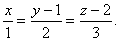Q. 25. Solve the following differential equation: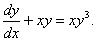Q. 26. Two balls are projected from the same point in direction included at 60 0 and 30 0 to the horizontal.
(i) If they attain the same height, what is the ratio of their velocities of projection?
(ii) If they have the same horizontal range, what is the ratio of their velocities of projection?

Or

A ball is projected vertically upwards with a velocity of 19.6 m/s. Find the
(i) Time taken to reach the greatest height.
(ii) The greatest height reached.
(ii) The position of the ball after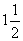seconds. [Use g = 9.8 m/s 2 ]

SECTION - C

Q. 19. The first four moments of distribution about the value 5 of the variable are 2, 20, 40 and 50 respectively. Find the
(i) Mean
(ii) Variance
(iii)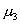Ff
Of the distribution.

Q. 20. For a near normal distribution, find Bowley's co-efficient of skewness for which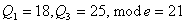and mean = 18.

Q. 21. A, B and C are partners in a business. A invests ¼ of the capital for ¼ of the time, B, 1/5 th of the capital for ½ of the time and C the remainder of the capital for the whole time. Find the shares in a profit of Rs. 34,200.

Q. 22. A loan of Rs. 40,000 is repaid at 5% per annum compound interest in equal annual instalments beginning at the end of first year. Find the amount of each instalment. [Take (1.05) -10 = 0.6138]

Q. 23. Three are two identical boxes containing respectively 4 white and 3 red balls, 3 white and 7 red balls. A box is chosen at random and a ball is drawn from it. It the ball drawn is white, what is the probability that it is from the first box?

Q. 24. A manufacture of bulbs knows that on an average 5% of his production is defective. He sells bulbs in boxes of 100 pieces and guarantees that not more than 4 bulbs will be defective in a box. What is probability that a box will meet the guarantee? (Use e -5 = 0.0067)

Q. 25. The cost function of a firm is given by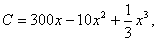Where x is the output. Find the output at which (i) the average cost is minimum, (ii) the average cost is equal to the marginal cost.

Q. 26. A dealer wishes to purchase a number of fans and sewing machines. He has only Rs. 5,760 to invest and has space for at the most 20 items. A fan costs him Rs. 360 and a sewing machine Rs. 240. He expects to sell a fan at a profit of Rs. 22 and a sewing machine for a profit of Rs. 18. Assuming that he can sell all the items that he buys, how should he invest his money to maximum his profit? Solve it graphically.

Or

A company sells two different produces A and B. The two products are produced in a common production process which has a total capacity of 500 man hours. It takes 5 hours to produce a unit of A and 3 hours to produce a unit of B. The demand in the market shoes that he maximum number of units of A that the maximum number of units of A that can be sold is 70 and that for B is 125. Profit on each unit of A is Rs. 20 and that on B is Rs. 15. How many units of A and B should be produced to maximize the profit? Solve it graphically.

 Mathematics 2003 Question Papers Class XII Delhi Outside Delhi Compartment Delhi Compartment Outside DelhiSet ISet ISet ISet ISet IISet IISet IISet IISet IIISet III

CBSE 2003 Question Papers Class XII